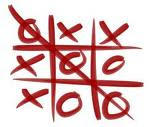### Tips & Tricks for Teaching MultiplicationLearning multiplication doesn't have to be like in the comic pictured below but it can be lots of FUN!by Todd Wilson at Family Man Ministries
Here are some simple tips to help your child learn the multiplication facts. Begin teaching multiplication by helping your child understand how it relates to addition. Using an egg crate is an example of a fantastic way to help your child sort small objects, such as beans, into groups.I also like using Cuisenaire Rods, which I explain in this post, as a fun way to help children better understand the concept of multiplication.

Here are some fun tricks:
• Any number times zero is always zero.
• Any number times one is always itself.
• Double the number when multiplying by twos.
• Count by fives when multiplying by fives.
• Count by tens or add a zero to the end of the number when multiplying by ten.
• 10 x 9 = 90
• Double the digit when multiplying by 11 (up to 99)
• 11 x 9 = 99
• Use these two FUN techniques when multiplying by nines.

(1) Use your fingers for learning the nine facts.Source

Watch this video to learn how. It's a super cool trick!

(2) Write the number patterns. First, write 0-9 straight down like this.
0
1
2
3
4
5
6
7
8
9

Next, start at the bottom and write 0-9 going up.
9
18
27
36
45
54
63
72
81
90

Now,  teach your child the pattern when multiplying by nines.

9= 9 x 1
18= 9 x 2
27= 9 x 3
36= 9 x 4
45= 9 x 5
54= 9 x 6
63= 9 x 7
72= 9 x 8
81= 9 x 8
90= 9 x 9

I love that trick! That is how I memorized the nine facts as a child.

I don't know why but there is one multiplication fact that consistently stumps many kids so I will remind them- 5,6,7,8

Using a Multiplication Table can also help children learn the number patterns.***If you child has a hard time memorizing the multiplication facts, read this post for more tips.***

After your child learns their facts, you can review the facts by playing Multiplication Bingo.

My kids enjoy playing the classic Tic-Tac-Toe game on-line for practicing math facts. It's also fun to play on Valentine's Day since the xoxo's can mean hugs and kisses.My kids are hooked on the classic squares game so I added this fun twist that I found on-line to our reviews.

My favorite multiplication game to play with my kids (and when I taught in the classroom) is played with dice and called Circles and Stars

2 x 3 = 6

Play multiplication games on-line such as these.

Of course there are a ton of fun apps to play on your devices to review multiplication facts. That is how my two youngest children review their facts on a daily basis.

To have the "MOST FUN EVER" with math, incorporate food (said by my kids).

Enjoy making multiplication fun!
Deborah from www.splitmultigradeclassroom.blogspot.com just shared in the comments below another great tip that I did not know! Have you ever noticed the pattern when you multiply 6 times any even single digit? For example 6 x 2 = 1/2 of 2 and repeat the two or 12, 6 x 4 = 1/2 of 4 and repeat the four or 24, 6 x 6 is 1/2 of 6 and repeat the 6 or 36, 6 x 8 is 1/2 of 8 and repeat the 8 or 48.

Thanks Deborah for sharing!